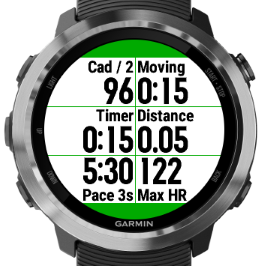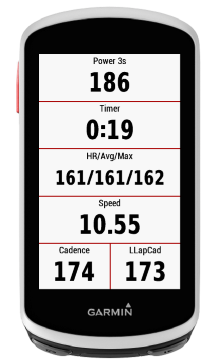# Data Field: AppBuilder

By user request, this app lets you define your own data field, based on a simple math formula like cadence / 2.

If you want to get fancy, there's conditional expressions (like IF in Excel), functions for data analysis (like MIN and AVG), and the data field can also display the text of your choice. The resulting data can be (optionally) written to the activity FIT file.

With AppBuilder, you can implement almost any field that involves math, such as: calculating normalized power and saving the data to the FIT activity file, showing the average pace for even-numbered laps, or recording the amount of time you sprinted.

Full documentation and examples here:
http://ciq-appbuilder.blogspot.com/

AppBuilder 5:
Now with new features! AppBuilder 5 is a completely new app, so please check it out in the store if you are interested in any of the new features.
https://apps.garmin.com/en-US/apps/fd690281-9c22-4fee-a81e-3b7f39aa67c5

- Store up to four formulas per app. Switch between formulas directly on the watch, without using a phone or PC. With four clone apps, you can store up to 16 formulas total
- User variables. Allows for powerful formulas where information can be stored at one point, like the start of a lap, and used later. e.g. Lap elevation change
- Improved timeavg() options. Allows for simpler, more flexible normalized power function
- More functions and variables

4 clones of AppBuilder are available in the store, so you can have 2 formulas in the same activity
• Documentation and examples:
http://ciq-appbuilder.blogspot.com/

AppBuilder 5+ screenshots:• Nice to see I've peaked your interest level :)
enjoy the project ;)
• Thanks for the motivation! :) TBH I have no idea if anyone will even want to use an app like this.
• The app has been updated:
- Added additional activity data: power, altitude, total ascent, total descent, training effect, and energy expenditure
- Changed the relational operators, so you can actually configure them through Garmin Connect
• Can you add averaging on time value something like average speed for 10 s
Thank You for hard work
• Can you add averaging on time value something like average speed for 10 s
Thank You for hard work

No worries! :) I'm actually surprised nobody has ever done this before. I assumed that nobody would want it. (Time will tell, I guess.)

The latest update (2.2.1) has a couple of new functions:

TIMEAVG(value, t) Calculates the average of value over the last t seconds (one sample per second).
MIN(value) Calculates the minimum of value over the entire activity
MAX(value) Calculates the maximum of value over the entire activity

I added these functions for CIQ 2 devices only. Unfortunately, no support on 230, 235 ,630, Fenix 3, 920XT, Vivoactive or D2.

That's a great idea! Thanks. I don't think there's a native value for elevation grade, but we can calculate it.

I defined a function PREV(x) which returns the last sampled value of x (from 1 second ago).

You can calculate the gradient as follows:
(altitude_raw - PREV(altitude_raw)) / (distance_raw - PREV(distance_raw)) * 100

The 30s average (for example) would be:
TIMEAVG((altitude_raw - PREV(altitude_raw)) / (distance_raw - PREV(distance_raw)),30) * 100

---

Edit:
As easuvorov pointed out, the two formulas above don't provide the best results.

I did implement a better way to calculate the gradient (over the last 100m), but I haven't tested it in real life (yet). You should be able to use this formula:

(altitude_raw - PREVD(altitude_raw, 100)) / (distance_raw - PREVD(distance_raw, 100)) * 100

(Thanks easuvorov!)

Also, some watches have a native field for grade, but it isn't available to apps afaik.
• I defined a function PREV(x) which returns the last sampled value of x (from 1 second ago).

You can calculate the gradient as follows:
(altitude_raw - PREV(altitude_raw)) / (distance_raw - PREV(distance_raw)) * 100

The 30s average (for example) would be:
TIMEAVG((altitude_raw - PREV(altitude_raw)) / (distance_raw - PREV(distance_raw)),30) * 100

Unfortunately for us developers, gradient calculation is not that simple: to calculate gradient in it's simplest form you need altitude difference divided by distance difference that's right, but this should be calculated over a distance difference around 100 meters for a value to have any practical meaning, averaging of previous second differences over a period of time will give you mathematically different result, I'm afraid.
• Yeah, I sort of figured. Obviously runners especially aren't moving fast enough for the 1s deltas to mean much.

In an app like this, it's probably the best I can do, unless I add it as a built-in metric. I'll think about it. Thanks!

I would really prefer to not have any built-in custom metrics that could conceivably be defined using generic functions. (Or any built-in custom metrics at all).

Maybe a couple of fixed functions might help: